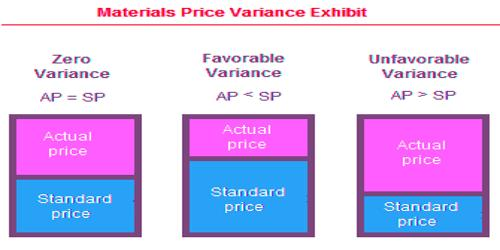# Material Price Variance

Material Price Variance

Direct Material Price Variance is the difference between the actual cost of direct material and the standard cost of quantity purchased or consumed. The variance is used to spot instances in which a business may be overpaying for raw materials and components. It refers to the variance that arises due to the dissimilarity in the actual and standard purchase price of raw materials used in production.

Direct Material Price Variance:

[Actual Quantity x Standard Price] – [Actual Quantity x Actual Price]

It is calculated using the following formula:

DM Price Variance = ( SP − AP ) × AQ

Where,

• SP is the standard unit price of direct material
• AP is the actual price per unit of direct material
• AQ is the actual quantity of direct material used

Where:

• Actual Quantity is the quantity purchased during a period if the variance is calculated at the time of material purchase,
• Actual Quantity is the quantity consumed during a period if the variance is calculated at the time of material consumption.

A positive value means that direct material was purchased for a smaller amount than the normal price. A negative value is critical because more than a predictable price per unit is paid.

The direct material price variance is one of the two variances used to observe direct materials. The other variance is the direct material yield variance. Thus, the price variance tracks differences in raw material prices, and yield variance tracks differences in the number of raw materials used.